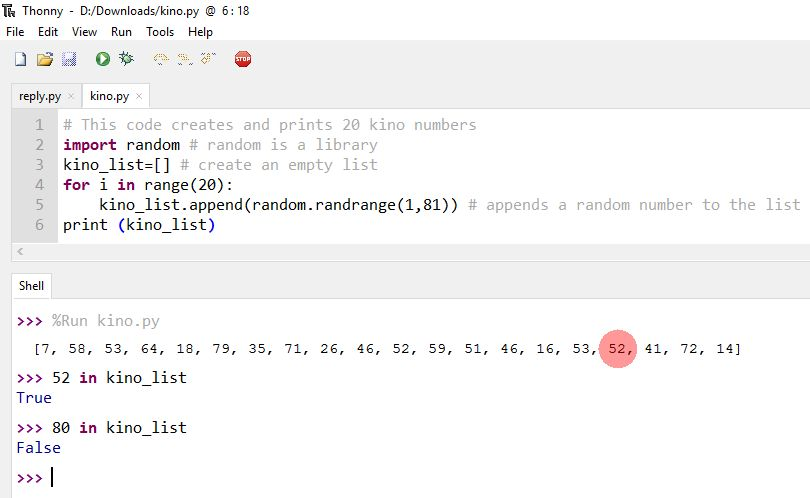# Week 3 - Section 2 - Graphics

## Chapter 5.1 - What is in a list?

When we created a list of 20 different numbers (kino) in previous lesson (Chapter 4.4) we asked you if the numbers in the list were unique. The answer is NO because when we add random numbers we can add more than once the same number.

So if you have saved the kino program open it or else go to chapter 4.4,  copy the code from there and save it as kino.

After executing the code look at the output find a number and write the commands as in the picture.With in command we can search inside a list.

Copy and paste the following code to Thonny and save it as kino2 :

# This code creates and prints 20 Unique kino numbers import random # random is a library kino_list=[] # create an empty list for i in range(20): new_number = random.randrange(1,81) while new_number in kino_list: # if new number is in the list select another until new_number = random.randrange(1,81) # it is not in the list kino_list.append(random.randrange(1,81)) # appends a random number to the list print (kino_list)

Compare it with the previous code of kino program. Are there 20 unique numbers now?